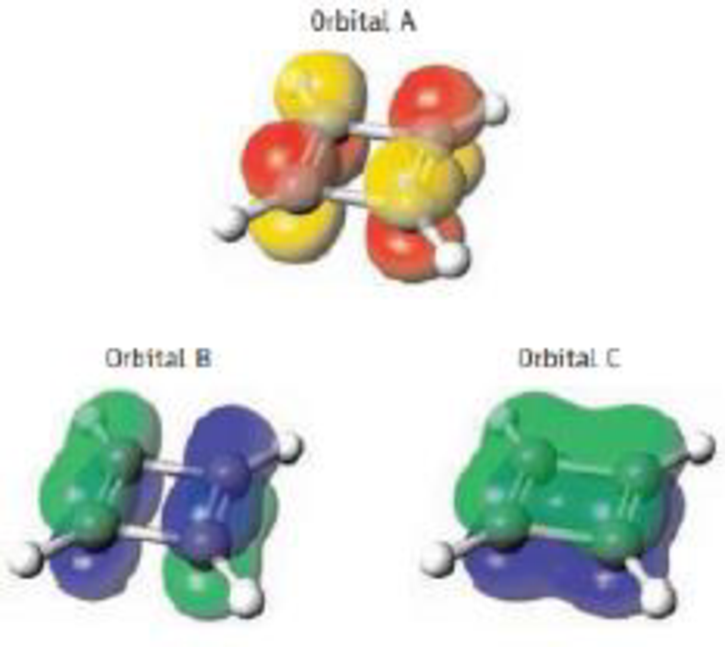Chapter 9, Problem 65SCQ

Chapter
Section
Textbook Problem

Three of the four π molecular orbitals for cyclobutadiene are pictured here. Place them in order of increasing energy. (See Figures 9.13, 9.15, 9.16, and 9.18 and the relation of orbital energy and nodes.)Interpretation Introduction

Interpretation:

The increasing order of orbitals nodes has to be explained.

Concept Introduction

Orbital nodes: This concept explain for number of nodes is always one less than the principle quantum number, the nodes indicated for (n=-1). The s-orbital in the first electron shell n=1, so 1s orbital has no nodes.

According to Molecular orbital (MO) theory,

The atomic orbitals of the atoms constituted in a molecule are combined to produce new orbitals are called Molecular Orbitals.

Like atomic orbitals, a molecular orbital can accommodate maximum two electrons and the two electrons must have opposite spins (Pauli Exclusion Principle).

The numbers of MO’s are equals to the number of atomic orbitals are combined in such a way that the linear combination of similar atomic orbitals to form one bonding and one anti-bonding MO’s.

The bonding MO’s are lower in energy than the anti-bonding MO’s

Energy of molecular orbitals is,

Anti-bonding molecular orbital > Non-bonding molecular orbital >Bonding molecular orbital

Explanation

In orbital A, red and yellow corresponds to the unoccupied carbon atom. So this is the anti-bonding molecular orbital and thus has the highest energy.

In orbital B The corner atoms are partially occupied molecular orbital. It implies that this is the non-bonding molecular orbital and thus has the lowest energy

Still sussing out bartleby?

Check out a sample textbook solution.

See a sample solution

The Solution to Your Study Problems

Bartleby provides explanations to thousands of textbook problems written by our experts, many with advanced degrees!

Get Started